C语言编程入门之函数传递指针参数的问题详解C语言函数传递指针参数的问题

一个问题是，我们想用一个函数来对函数外的变量v进行操作，比如，我想在函数里稍微改变一下这个变量v的值，我们应该怎么做呢？又或者一个常见的例子，我想利用swap()函数交换两个变量a,b的值，我们应该怎么做呢（好吧，博主是觉得这个问题十分经典）。

C语言里，改变值只能通过指针（地址）方式进行传递，或许你会说传递数组不是也可以改变值么，实际上，传递数组就是传递指针（或许对数组来说，这个指针有点特别）//注意：C里没有引用，C++里才有

code case 1

#include <stdio.h>

void swap(int a,int b)

{

int temp=a;

a=b;

b=temp;

}

int main()

{

int a=4,b=5;

swap(a,b);

printf("a = %d ,b = %d\n",a,b);

return 0;

}</stdio.h>

code case 2

#include <stdio.h>

void swap(int a,int b)

{

int temp=a;

a=b;

b=temp;

}

int main()

{

int a=4,b=5;

swap(a,b);

printf("a = %d ,b = %d\n",a,b);

return 0;

}</stdio.h>

a = 4 ,b = 5

code case 3

#include <stdio.h>

void swap(int *a,int *b)

{

int temp=*a;

*a=*b;

*b=temp;

}

int main()

{

int a=4,b=5;

swap(&a,&b);

printf("a = %d ,b = %d\n",a,b);

return 0;

}</stdio.h>

a = 5 ,b = 4

code case 4

#include <stdio.h>

void swap(int *a,int *b)

{

printf("address in swap(),the value of a and b:%p %p\n",a,b);

int temp=*a;

*a=*b;

*b=temp;

}

int main()

{

int a=4,b=5;

swap(&a,&b);

printf("a = %d ,b = %d\n",a,b);

return 0;

}</stdio.h>

address in swap(),the value of a and b:0061FF2C 0061FF28

a = 5 ,b = 4

#include <stdio.h>

#include <malloc.h>

typedef struct LNode

{

int data;

struct LNode *next;

}LNode;

{

L=(LNode *)malloc(sizeof(LNode));

L->data=0;

L->next=NULL;

}

int main()

{

LNode *L=NULL;

printf("%p\n",L);

return 0;

}</malloc.h></stdio.h>

#include <stdio.h>

#include <malloc.h>

typedef struct LNode

{

int data;

struct LNode *next;

}LNode;

{

L=(LNode *)malloc(sizeof(LNode));

L->data=0;

L->next=NULL;

return L;

}

int main()

{

LNode *L=NULL;

printf("%p\n",L);

return 0;

}</malloc.h></stdio.h>

006D1588

#include <stdio.h>

#include <malloc.h>

typedef struct LNode

{

int data;

struct LNode *next;

}LNode;

{

(*L)=(LNode *)malloc(sizeof(LNode));

(*L)->data=0;

(*L)->next=NULL;

}

int main()

{

LNode *L=NULL;

printf("%p\n",L);

return 0;

}</malloc.h></stdio.h>

006B1588

（注：采用此种方式是及其复杂的，因为这是一个二级指针，会增加理解难度，所幸的是，c++中的引用，可以避免这个问题）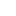喜欢 | 0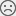不喜欢 | 0

15以上年开发与技术培训经验

• 10
文章
• 914
人气
• 100%
受欢迎度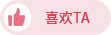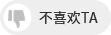•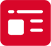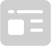索取资料
•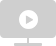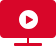答疑解惑
•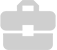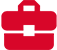技术交流
•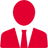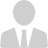职业测评
•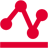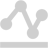面试技巧
•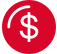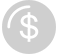高薪秘笈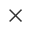Java全栈开发
WEB前端+H5沪公网安备 31011502005948号    ICP许可  沪B2-20190160